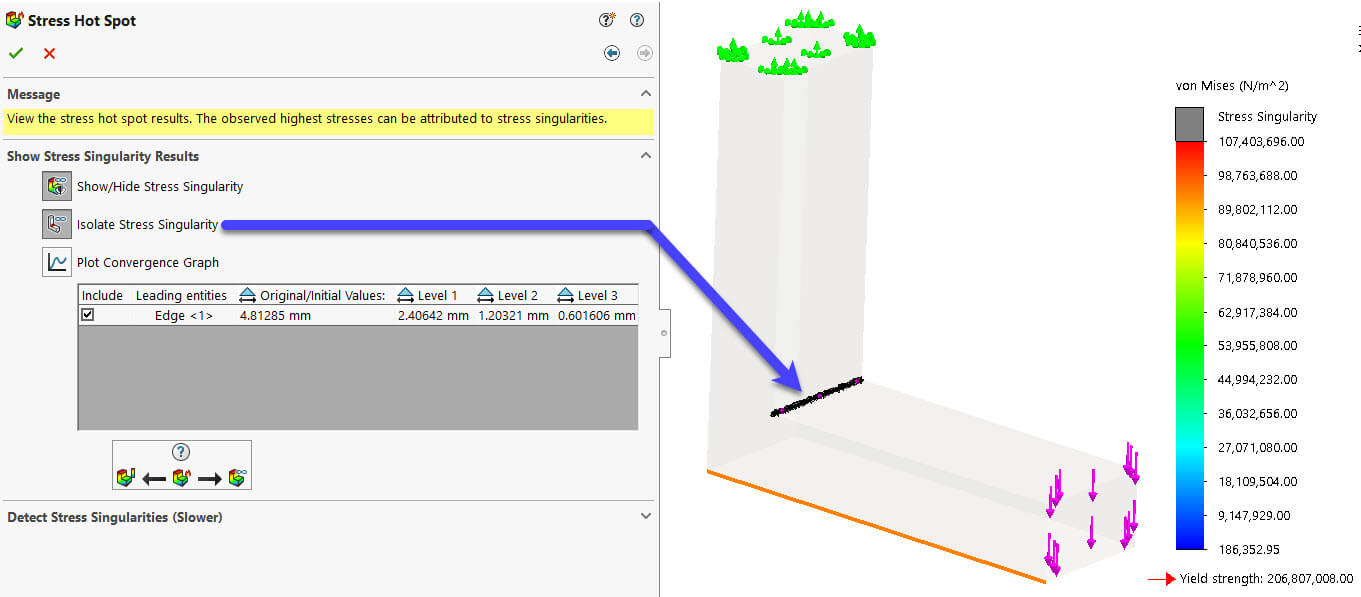# SOLIDWORKS Simulation 2018: Mesh Refinement for Stress Singularities Hot Spot DiagnosticArticle by Siavash Khajehhasani updated January 5, 2018

###### Article

The Stress Hot Spot Diagnostic Tool introduced in SOLIDWORKS Simulation 2017 helps engineers find hot spot regions in their model and possibly look for a potential ‘stress singularity’. In SOLIDWORKS 2018, this process has been enhanced to reduce the manual mesh refinement process in order to determine if the stress is real, or if it is a singularity.

## Stress Singularity

Not all stress hot spots are associated with stress singularities. Refining locally the mesh at hot spot regions can eliminate these stress hot spots attributed to stress concentrations that are non-singular. A characteristic behavior of a stress singularity is that the stress value keeps increasing with mesh refinement (either locally using mesh controls or globally by editing the mesh), and is diverging theoretically to infinity. If the stresses at the elements of stress hot spots do not increase with mesh refinement and converge towards a finite value, then they are indicative of stress concentrations (areas of ‘legitimate’ high stresses).Figure 1. Stress Singularity

Diverging stresses near sharp re-entrant corner as the mesh gets refined are indicative of stress singularities.

### Stress Concentration

Stress concentrations are characterized by relatively large stress gradients over adjacent elements at a localized area of a model. High stress gradients attributed to legitimate stress concentrations will converge to a limit value given that the mesh is sufficiently refined. They are visible where there are changes in geometry, such as a presence of a hole in a plate, at boundaries where different materials are present, and at points where bodies come into contact.Figure 2. Stress Concentration

Converging stresses near the fillet areas as the mesh gets refined are indicative of stress concentrations.

### Stress Hot Spot Diagnostic

The ‘stress hot spot diagnostic’ tool can be accessed by right-clicking on results folder. In the first menu, the sensitivity factor as well as if node values results are also needed to be processed, can be set up, which were also available in 2017, as shown in Figure 3.Figure 3. Stress Hot Spot Diagnostic (sensitivity factor setting)

### Stress Singularity Diagnosis

Now in SOLIDWORKS Simulation 2018, stress singularity detection tool is added. Wherein, the target area(s) such as a sharp edge will be selected and then user can specify how many mesh refinement levels should be taken. Please note that the maximum mesh refinement level is 3. Also the element size reduction factor as well as element growth ratio can be specified.

Use can also decides if the final results should be displayed based on the original mesh resolution of the final mesh density based of the final mesh refinement level. In the current example, the final mesh refinement is shown as depicted in Figure 6.Figure 4. Stress Singularity Diagnosis

If there singularity is being detected, as described before, then the Stress Singularity Diagnosis tool shows the following message to inform the user.Figure 5. Stress Singularities Detection message

Thereafter, user can isolate the elements which are having highest stress values based on the sensitivity factor (herein 25%), as shown in Figure 6.Figure 6. Isolated Elements

Finally, ‘Plot Convergence Graph’ demonstrates the trend of stress values for each level of refinement (Figure. 7).Figure 7. Plot Convergence Graph

### Recommendations on Treating Stress Singularities

• To differentiate stress singularities from legitimate stress concentrations, refine the mesh either globally, or locally at the regions where stress hot spots are detected. When you rerun the stress hot spot diagnostics tool, the areas of stress concentrations should be filtered out, and the remaining stress hot spots would point to stress singularities. Note that in the physical world, stress singularities do not occur as the material will yield or crack when stresses exceed the material’s ultimate strength limit.
• Add fillets to sharp edges and “round off” corners where high stress gradients occur. The fillets distribute the stress over a broader area and effectively increase the load bearing capacity of parts. Remember that in the real world a manufactured “sharp corner” will always have a small fillet radius.
• Avoid applying loads on vertices or along edges, if you can. Distribute the load to the proper tributary areas on the model to approximate as accurately as possible the real loading conditions. Remember that even if the load is applied as a singular point load creating a stress singularity (σ = P/A and A=0 → σ=∞), the stress distribution some distance away from the applied load will still be correct. This is based on the St. Venant’s principle that states that the difference between the effects of two different but statically equivalent loads becomes very small at sufficiently large distances from the load.
• Stress singularities can pollute stress distributions only locally. You may ignore these localized areas of stress singularities, especially if they are not near the area of interest in your simulation, and examine the stress results further away which should remain “unpolluted” .
###### References:

. 2018 SOLIDWORKS help document, assessing stress hot spots.